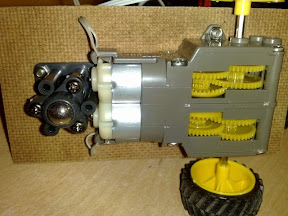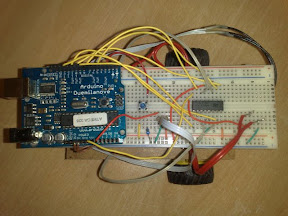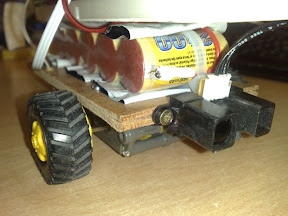# MiniBot

simple and cheap Arduino Robot

Code and some detailed information after the break

The Bot is build with the Tamiya Twin Motor Gearbox, Tamiya Truck Tire´s and
the Tamiya Ball Caster. All mounted on a piece of plywood.

They can be found at Sparkfun´s Robotics section: http://www.sparkfun.com/Stuck on top is a old battery pack and a breadboard with the Arduino and the L293D Motordriver.Also a GP2Y0A02YK Distance sensor sits on the plywood, professionaly mounted with too big screws# Code

As simple as the Robot is the Code.

It watches if something gets to close, and then turns until it finds no obstacle.
Then it moves until something gets to close, and so on...

Arduino code:

`// constants won't change. They're used here to // set pin numbers:const int buttonPin = 2;     // the number of the pushbutton pinconst int ledPin =  13;      // the number of the LED pinconst int M1pwm = 9;const int M1a = 4;const int M1b = 7;const int M2pwm = 10;const int M2a = 8;const int M2b = 12;int Dist = 0;// variables will change:int buttonState = 0;         // variable for reading the pushbutton statusvoid setup() {  // initialize the Outputs:  pinMode(ledPin, OUTPUT);    pinMode(M1a, OUTPUT);    pinMode(M1b, OUTPUT);    pinMode(M2a, OUTPUT);    pinMode(M2b, OUTPUT);      // initialize the pushbutton pin as an input:  pinMode(buttonPin, INPUT);  }void loop(){  // read the state of the pushbutton value:  buttonState = digitalRead(buttonPin);  if (buttonState == HIGH) {         // turn LED on:  and Motor on       digitalWrite(ledPin, HIGH);      //Fetch the Analog Signal:        Dist = analogRead(0);        //The Bot should turn until it finds a spot where it can travel more as 50cm.     //50cm is about 1.5V.            LoopSpot:        Turn(60,true);        do    {     Dist = Dist * 0.5 + analogRead(0) * 0.5;    }while (Dist > 400);       Straight(0,true);    delay(100);    //Now it can move         Straight(80,true);        //But only until it find another stop)        do    {     Dist = Dist * 0.5 + analogRead(0) * 0.5;    }while (Dist < 400);            //Now we only stop, but this could be endless        Straight(0,true);    delay(100);        goto LoopSpot;          }   else {    // turn LED off:    digitalWrite(ledPin, LOW);         Straight(0,true);          }}void Straight(int Speed, boolean forward){  //This drives the bot Straight forward or reverse    if(forward==true)  {    digitalWrite(M1a, HIGH);      digitalWrite(M1b, LOW);          digitalWrite(M2a, HIGH);      digitalWrite(M2b, LOW);    }  else  {    digitalWrite(M1a, LOW);      digitalWrite(M1b, HIGH);          digitalWrite(M2a, LOW);      digitalWrite(M2b, HIGH);    }    analogWrite(M1pwm,Speed);  analogWrite(M2pwm,Speed);    }void Turn(int Speed, boolean right){  //This drives the bot right or left  //it is a spin on its position, so the wheels turn the other way   if(right==true)  {    digitalWrite(M1a, HIGH);      digitalWrite(M1b, LOW);          digitalWrite(M2a, LOW);      digitalWrite(M2b, HIGH);    }  else  {    digitalWrite(M1a, LOW);      digitalWrite(M1b, HIGH);          digitalWrite(M2a, HIGH);      digitalWrite(M2b, LOW);    }    analogWrite(M1pwm,Speed);  analogWrite(M2pwm,Speed);   }  `